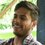# Help in Solving Physics Differential equation.

Hello everyone!

I'm unable to solve this differential equation while solving a physics question. So please help me in solving this equation:

$m\frac{dv}{dt}=mg -\lambda\frac{v}{x(x-d)}$ or

$m\frac{vdv}{dx}=mg-\lambda \frac {v }{x(x-d)}.$

$UPDATE$:

$Here$ $I$ $want$ $to$ $express$ $either$

$v=f(t)$

or

$v=f(x)$.

$Given$.

where all terms have the usual meaning:

'$\lambda$' is constant.

$v$ is the velocity of the rod (variable)

$m$ is the mass of the rod (constant)

$x$ is the distance from a fixed point to the rod (variable)

$t$ is time (obviously a variable)

and $d$ is the intial distance (constant)

$Note$:

This is a part of a question that I'm writing. I'm unable to proceed further because of this difficult differential equation.

UPDATENote by Deepanshu Gupta
6 years, 9 months ago

This discussion board is a place to discuss our Daily Challenges and the math and science related to those challenges. Explanations are more than just a solution — they should explain the steps and thinking strategies that you used to obtain the solution. Comments should further the discussion of math and science.

When posting on Brilliant:

• Use the emojis to react to an explanation, whether you're congratulating a job well done , or just really confused .
• Ask specific questions about the challenge or the steps in somebody's explanation. Well-posed questions can add a lot to the discussion, but posting "I don't understand!" doesn't help anyone.
• Try to contribute something new to the discussion, whether it is an extension, generalization or other idea related to the challenge.

MarkdownAppears as
*italics* or _italics_ italics
**bold** or __bold__ bold
- bulleted- list
• bulleted
• list
1. numbered2. list
1. numbered
2. list
Note: you must add a full line of space before and after lists for them to show up correctly
paragraph 1paragraph 2

paragraph 1

paragraph 2

[example link](https://brilliant.org)example link
> This is a quote
This is a quote
    # I indented these lines
# 4 spaces, and now they show
# up as a code block.

print "hello world"
# I indented these lines
# 4 spaces, and now they show
# up as a code block.

print "hello world"
MathAppears as
Remember to wrap math in $$ ... $$ or $ ... $ to ensure proper formatting.
2 \times 3 $2 \times 3$
2^{34} $2^{34}$
a_{i-1} $a_{i-1}$
\frac{2}{3} $\frac{2}{3}$
\sqrt{2} $\sqrt{2}$
\sum_{i=1}^3 $\sum_{i=1}^3$
\sin \theta $\sin \theta$
\boxed{123} $\boxed{123}$

Sort by:

In the first equation multiply both sides by differential $dt$.Also write $v = \frac {dx}{dt}$.

You should get : $mdv = mgdt - \frac {\lambda dx}{x(x-d)}$

You also need to know three initial conditions : Initial time which is $0$,initial position and initial velocity.

Did I make a mistake in doing this?

- 6 years, 9 months ago

Yes i did it in same way...!!

But I want to express velocity as either $v=f(t)$ or $v=f(x)$ so that further question is completed. Since Both time and distance are different. I mean to say if I want to calculate say velocity at the instant say at t=10 s then how could I know velocity at that instant since position is also unkown at that instant of Time. ?? and Also according to this question $v = \frac {d(x-d)}{dt}$. which is also same thing as $v = \frac {dx}{dt}$.

- 6 years, 9 months ago

I believe the differential equation is not meant to be solved by wolfram alpha itself.

- 6 years, 9 months ago

@Ronak Agarwal @jatin yadav will you help ??

- 6 years, 9 months ago

@Pranav Arora @Finn Hulse any Help??

- 6 years, 9 months ago

I don't think I'll be of much help on the problem, but certainly the LaTeX needed editing. Is this the way you would like it to appear?

- 6 years, 9 months ago

Thanks,@Finn But how can I expressed $v=f(x)$ or $v=f(t)$.?? which is essential for this question. ( that is my Problem)

- 6 years, 9 months ago

I'm not sure I understand what you mean.

- 6 years, 9 months ago

Is it possible to express the velocity as an individual function ?? or we require Higher mathamatics??

- 6 years, 9 months ago

Are you asking if you can solve for $v$? Because if you are, I think it would be very tricky.

- 6 years, 9 months ago

yes.. will you please give a try to it ? I make all possible attempt but not succesfull in it.

- 6 years, 9 months ago

Sure. Also, I've been to Jaipur! It's super awesome! :D

- 6 years, 9 months ago

wow.. !! A very warm welcome to you for coming in Jaipur..... In our Language we says:

"$padharo$ $mhare$ $desh$"

Well for what purpose you have been come here... is it Vacation's celebration or something else ?

- 6 years, 9 months ago

I took a trip around Rajasthan and also to Agra (for obvious reasons). I visited Delhi, Jaipur, Udaipur, Bundi, Chittogarth, Ranthambhore, and some other places. :D

- 6 years, 9 months ago

@Calvin Lin sir @Michael Mendrin sir any help??

- 6 years, 9 months ago

Can you please post that whole physics question itself on which you are working.

- 6 years, 9 months ago

@Ronak Agarwal Ok Done it. You can check it Here.

- 6 years, 9 months ago

Hi Deepanshu please try this question. I think you will find this interesting.

- 6 years, 9 months ago

@satvik pandey Done..!!

- 6 years, 9 months ago